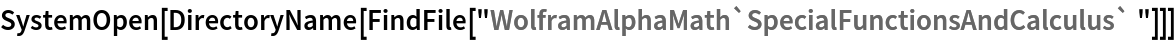Function Repository Resource:

# FractionalOrderD

Compute the fractional derivative of an expression

Contributed by: Oleg Marichev & Paco Jain (Wolfram Research)
 ResourceFunction["FractionalOrderD"][f,{x,α}] gives the α-order fractional integro-derivative of f with respect to x.

## Details

There are different ways of defining the fractional integro-derivative. The current implementation uses the Riemann-Liouville formulation, defined by: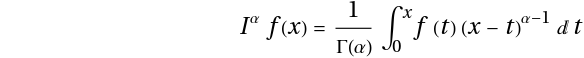ResourceFunction["FractionalOrderD"] automatically maps over lists in its first argument.

## Examples

### Basic Examples (5)

Compute a fractional derivative of a power function:

 In:=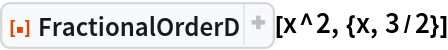Out=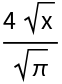The first-order fractional derivative is equivalent to differentiation:

 In:=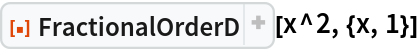Out=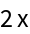In:=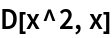Out=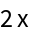The negative-first-order fractional derivative is equivalent to indefinite integration:

 In:=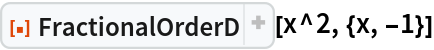Out=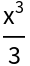In:=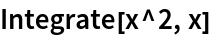Out=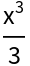Compute the fractional derivative of symbolic order α of an exponential function:

 In:=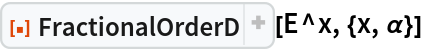Out=Compute the fractional derivative of symbolic order α of a logarithmic function:

 In:=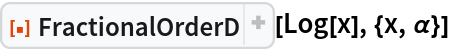Out=### Scope (1)

FractionalOrderD works for both numeric and symbolic orders:

 In:=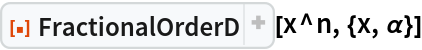Out=In:=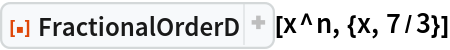Out=### Possible Issues (2)

FractionalOrderD may return results in terms of inactivated symbols like Sum, Product, MeijerG, etc:

 In:=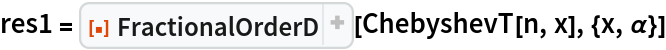Out=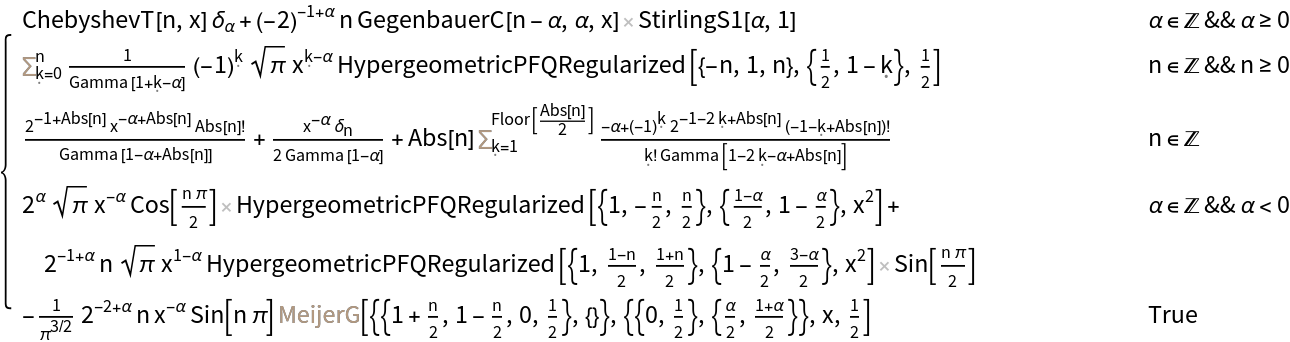In:=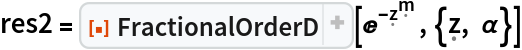Out=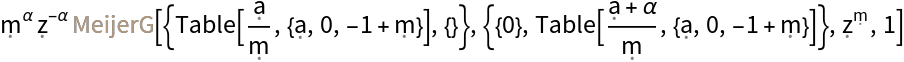In order to use such expressions, first substitute appropriate values of symbolic parameters and then apply Activate:

 In:=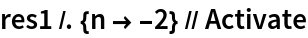Out=In:=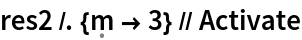Out=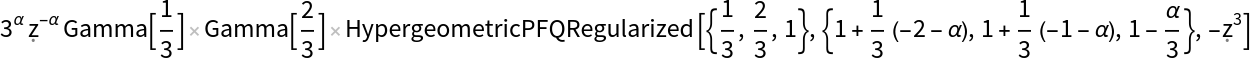In cases where only partial evaluation is possible, results may be returned that contain unevaluated FractionalOrderD expressions:

 In:=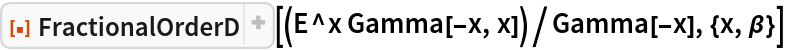Out=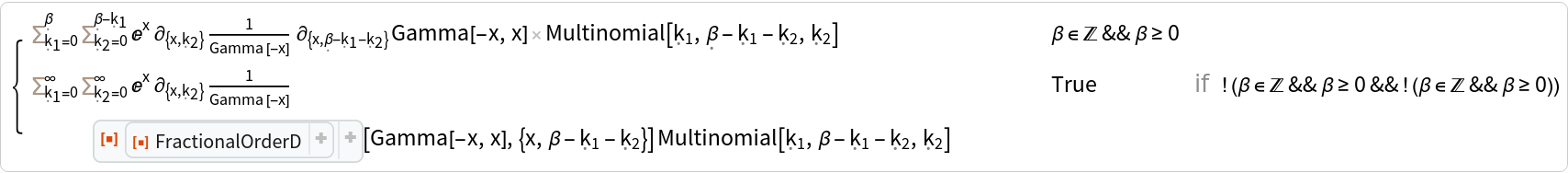### Interactive Examples (1)

Varying α from 0 to -1 smoothly interpolates between a function and its derivative:

 In:=Out=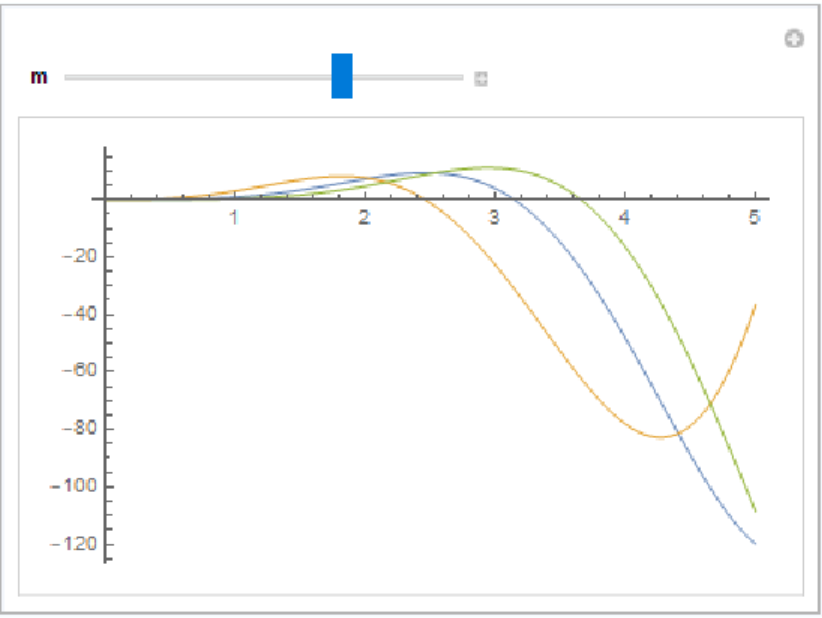### Neat Examples (1)

Create a table of fractional derivatives:

 In:=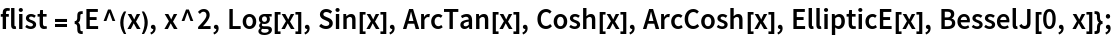In:=Out=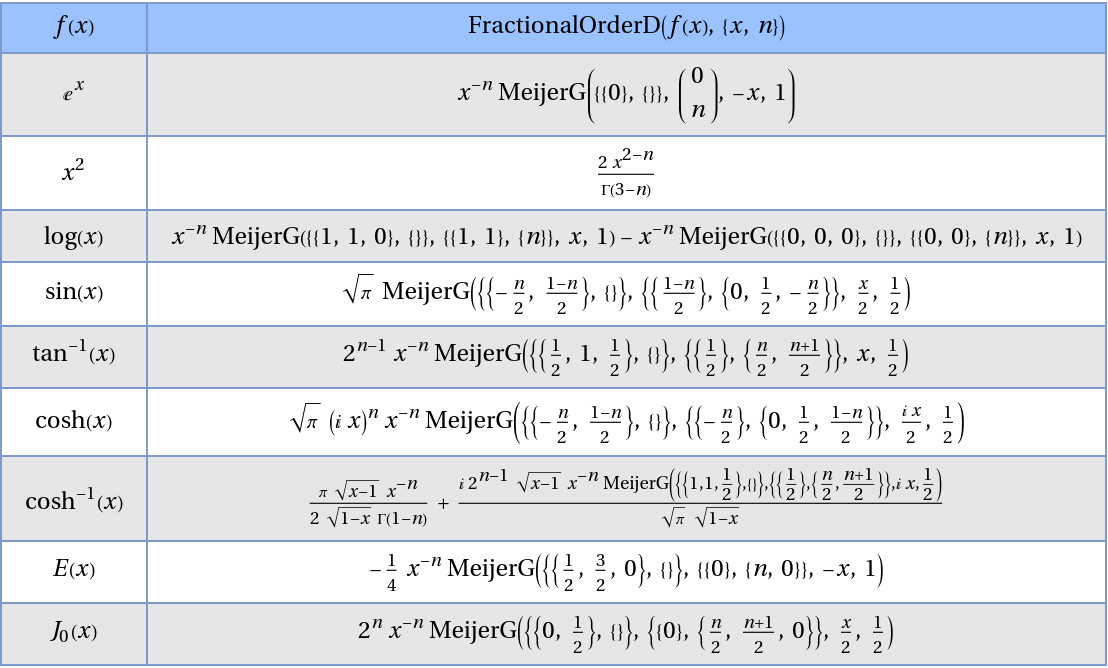## Publisher

Wolfram|Alpha Math Team

## Version History

• 3.2.0 – 14 August 2023
• 3.1.4 – 07 November 2022
• 3.1.3 – 11 October 2022
• 3.1.2 – 11 October 2022
• 3.1.1 – 23 August 2022
• 3.1.0 – 23 August 2022
• 3.0.0 – 23 August 2022
• 2.1.0 – 11 September 2021
• 2.0.0 – 05 October 2020
• 1.0.0 – 23 September 2020

## Author Notes

There are different ways of defining the fractional integro-derivative. The current implementation uses the Riemann-Liouville formulation. Another frequently-used definition, the Grunwald-Letnikov formulation may be implemented in a future version.

To view the full source code for this and related functions, evaluate the following:

 In:=